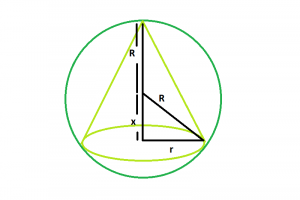# Largest right circular cone that can be inscribed within a sphere

Given sphere of radius. The task is to find the radius of base and height of the largest right circular cone that can be inscribed within it.

Examples:

```Input : R = 10
Output : r = 9.42809, h = 13.3333

Input : R = 25
Output : r = 23.5702, h = 33.3333

```

## Recommended: Please try your approach on {IDE} first, before moving on to the solution.Approach:

Let the radius of the cone = r
height of the cone = h

From the diagram it is clear that:
x = √(R^2 – r^2)
Now,

To maximize the volume of the cone(V):
V = (πr2h)/3

From the diagram,
V = (πr2R)/3 + πr2√(R2> – r2)/3

Taking derivative of V with respect to r we get,
dV/dr = 2πr(√(R2 – r2) + R)/3 – πr2√(R2 – r2)/3

Now, setting dV/dr = 0 we get,
r = 2√2R/3

since, h = R + √(R2 – r2)

So, h = 4R/3

Below is the implementation of the above approach:

## C++

 `// C++ Program to find the biggest cone ` `// that can be inscribed within a sphere ` `#include ` `using` `namespace` `std; ` ` `  `// Function to find the radius of the cone ` `float` `coner(``float` `R) ` `{ ` ` `  `    ``// radius cannot be negative ` `    ``if` `(R < 0) ` `        ``return` `-1; ` ` `  `    ``// radius of the cone ` `    ``float` `r = (2 * ``sqrt``(2) * R) / 3; ` `    ``return` `r; ` `} ` ` `  `// Function to find the height of the cone ` `float` `coneh(``float` `R) ` `{ ` ` `  `    ``// side cannot be negative ` `    ``if` `(R < 0) ` `        ``return` `-1; ` ` `  `    ``// height of the cone ` `    ``float` `h = (4 * R) / 3; ` `    ``return` `h; ` `} ` ` `  `// Driver code ` `int` `main() ` `{ ` `    ``float` `R = 10; ` ` `  `    ``cout << ``"r = "` `<< coner(R) << ``", "` `         ``<< ``"h = "` `<< coneh(R) << endl; ` ` `  `    ``return` `0; ` `} `

## Java

 `// Java Program to find the biggest cone ` `// that can be inscribed within a sphere ` `import` `java.util.*; ` `import` `java.lang.*; ` ` `  `class` `GFG ` `{ ` `// Function to find the radius ` `// of the cone ` `static` `float` `coner(``float` `R) ` `{ ` `    ``// radius cannot be negative ` `    ``if` `(R < ``0``) ` `        ``return` `-``1``; ` ` `  `    ``// radius of the cone ` `    ``float` `r = (``float``)(``2` `*  ` `            ``Math.sqrt(``2``) * R) / ``3``; ` `    ``return` `r; ` `} ` ` `  `// Function to find the  ` `// height of the cone ` `static` `float` `coneh(``float` `R) ` `{ ` ` `  `    ``// side cannot be negative ` `    ``if` `(R < ``0``) ` `        ``return` `-``1``; ` ` `  `    ``// height of the cone ` `    ``float` `h = (``4` `* R) / ``3``; ` `    ``return` `h; ` `} ` ` `  `// Driver code ` `public` `static` `void` `main(String args[]) ` `{ ` `    ``float` `R = ``10``; ` ` `  `    ``System.out.println(``"r = "` `+ coner(R) +  ` `                       ``", "` `+ ``"h = "` `+ coneh(R)); ` `} ` `} ` ` `  `// This code is contributed  ` `// by Akanksha Rai `

## Python3

 `# Python 3 Program to find the biggest cone  ` `# that can be inscribed within a sphere  ` `import` `math ` ` `  `# Function to find the radius  ` `# of the cone  ` `def` `coner(R): ` `     `  `    ``# radius cannot be negative  ` `    ``if` `(R < ``0``): ` `        ``return` `-``1``;  ` `     `  `    ``# radius of the cone  ` `    ``r ``=` `(``2` `*` `math.sqrt(``2``) ``*` `R) ``/` `3` `    ``return` `float``(r)  ` ` `  `# Function to find the height  ` `# of the cone  ` `def` `coneh(R): ` `     `  `    ``# side cannot be negative  ` `    ``if` `(R < ``0``): ` `        ``return` `-``1``;  ` ` `  `    ``# height of the cone  ` `    ``h ``=` `(``4` `*` `R) ``/` `3` `    ``return` `float``(h)  ` ` `  `# Driver code  ` `R ``=` `10` `print``(``"r = "` `, coner(R) ,  ` `      ``", "``, ``"h = "` `, coneh(R))  ` ` `  `# This code is contributed  ` `# by 29AjayKumar `

## C#

 `// C# Program to find the biggest cone ` `// that can be inscribed within a sphere ` `using` `System; ` ` `  `class` `GFG ` `{ ` `// Function to find the radius ` `// of the cone ` `static` `float` `coner(``float` `R) ` `{ ` `    ``// radius cannot be negative ` `    ``if` `(R < 0) ` `        ``return` `-1; ` ` `  `    ``// radius of the cone ` `    ``float` `r = (``float``)(2 *  ` `               ``Math.Sqrt(2) * R) / 3; ` `    ``return` `r; ` `} ` ` `  `// Function to find the  ` `// height of the cone ` `static` `float` `coneh(``float` `R) ` `{ ` ` `  `    ``// side cannot be negative ` `    ``if` `(R < 0) ` `        ``return` `-1; ` ` `  `    ``// height of the cone ` `    ``float` `h = (4 * R) / 3; ` `    ``return` `h; ` `} ` ` `  `// Driver code ` `public` `static` `void` `Main() ` `{ ` `    ``float` `R = 10; ` ` `  `    ``Console.WriteLine(``"r = "` `+ coner(R) +  ` `                      ``", "` `+ ``"h = "` `+ coneh(R)); ` `} ` `} ` ` `  `// This code is contributed  ` `// by Akanksha Rai `

## PHP

 ` `

Output:

```r = 9.42809, h = 13.3333
```

My Personal Notes arrow_drop_upCheck out this Author's contributed articles.

If you like GeeksforGeeks and would like to contribute, you can also write an article using contribute.geeksforgeeks.org or mail your article to contribute@geeksforgeeks.org. See your article appearing on the GeeksforGeeks main page and help other Geeks.

Please Improve this article if you find anything incorrect by clicking on the "Improve Article" button below.